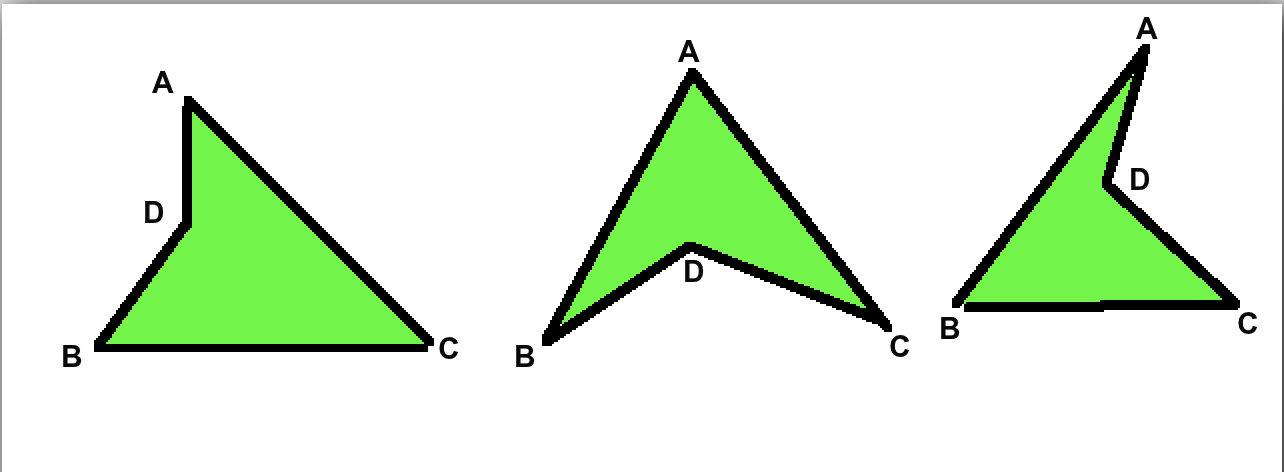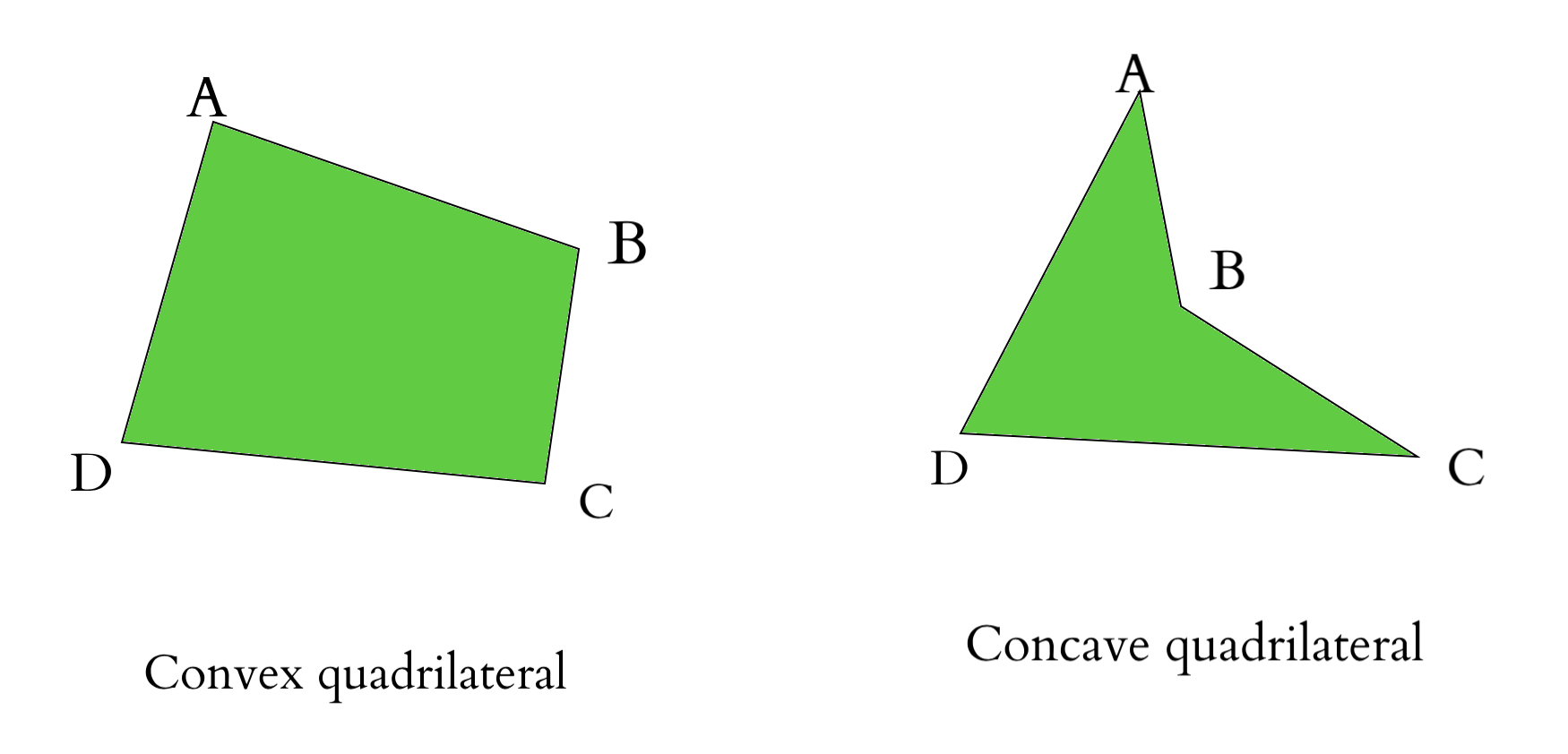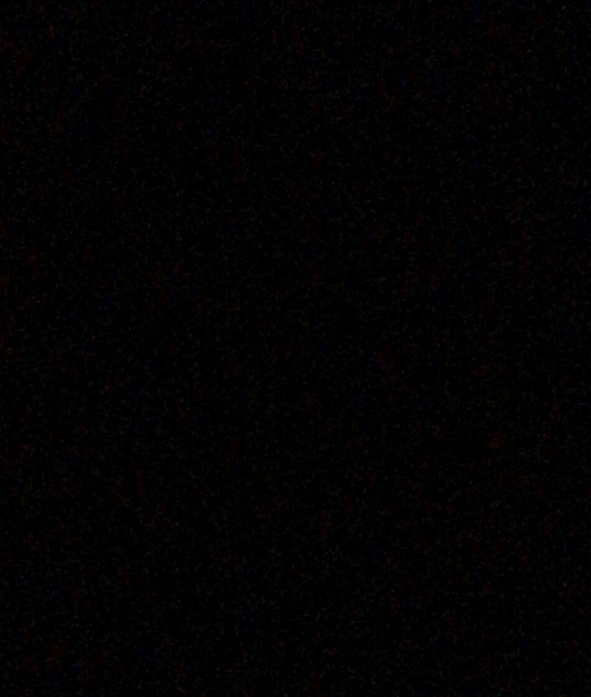# Number of quadrilaterals possible from the given points

Given four points (x, y) in the cartesian coordinate. Find the possible no of quadrilaterals than can be formed by joining all the four points.
Examples:

```Input: A=(0, 9), B=(-1, 0), C=(5, -1), D=(5, 9)
Output: Only one quadrilateral is possible (ABCD) in any orientation

Input: A=(0, 9), B=(-1, 0), C=(5, -1), D=(0, 3)
```

Figure for 2nd example:Approach:

1. We need to check whether any of the given points are same. If yes, no of quadrilateral = 0
2. Then we need to check whether any of the 3 points of the given 4 points are collinear or not. If yes, no of quadrilateral=0.Check Program to check if three points are collinear link to check collinearity of 3 points.
1. Now for a valid quadrilateral there are two possibilities:

2. if its a convex quadrilateral then there is only one possible quadrilateral.
3. if its a concave quadrilateral then there are 3 possible quadrilaterals.

This can be determined by How to check if two given line segments intersect? of diagonals.
In case of a convex quadrilateral, the diagonals will intersect whereas in case of a concave quadrilateral the diagonals won’t intersect.
since we don’t know the orientation of the points, we can’t specifically determine the diagonals so all the distinct line segments(no common points in the two line segments) of the quadrilateral and determine whether they intersect or not.

Refer to the figure to understand how to determine the type of quadrilateral :line AB and line DC do not intersect
line AD and line BC do not intersect
line AC and line BD intersect
so total no of intersection= 1

line AB and line DC do not intersect
line AD and line BC do not intersect
line AC and line BD do not intersect
so total no of intersection= 0

if no of intersection = 1, its a convex quadrilateral so no of possibility= 1
if no of intersection = 0, its a concave quadrilateral so no of possibilities = 3

## C++

 `// C++ implementation of above approach ` `#include ` `using` `namespace` `std; ` ` `  `struct` `Point ``// points ` `{ ` `    ``int` `x; ` `    ``int` `y; ` `}; ` ` `  `// determines the orientation of points ` `int` `orientation(Point p, Point q, Point r) ` `{ ` `    ``int` `val = (q.y - p.y) * (r.x - q.x) - (q.x - p.x) * (r.y - q.y); ` ` `  `    ``if` `(val == 0) ` `        ``return` `0; ` `    ``return` `(val > 0) ? 1 : 2; ` `} ` ` `  `// check whether the distinct line segments intersect ` `bool` `doIntersect(Point p1, Point q1, Point p2, Point q2) ` `{ ` `    ``int` `o1 = orientation(p1, q1, p2); ` `    ``int` `o2 = orientation(p1, q1, q2); ` `    ``int` `o3 = orientation(p2, q2, p1); ` `    ``int` `o4 = orientation(p2, q2, q1); ` ` `  `    ``if` `(o1 != o2 && o3 != o4) ` `        ``return` `true``; ` ` `  `    ``return` `false``; ` `} ` ` `  `// check if points overlap(similar) ` `bool` `similar(Point p1, Point p2) ` `{ ` ` `  `    ``// it is same, we are returning false because ` `    ``// quadrilateral is not possible in this case ` `    ``if` `(p1.x == p2.x && p1.y == p2.y) ` `        ``return` `false``; ` ` `  `    ``// it is not same, So there is a ` `    ``// possibility of a quadrilateral ` `    ``return` `true``; ` `} ` ` `  `// check for collinearity ` `bool` `collinear(Point p1, Point p2, Point p3) ` `{ ` `    ``int` `x1 = p1.x, y1 = p1.y; ` `    ``int` `x2 = p2.x, y2 = p2.y; ` `    ``int` `x3 = p3.x, y3 = p3.y; ` ` `  `    ``// it is collinear, we are returning false ` `    ``// because quadrilateral is not possible in this case ` `    ``if` `((y3 - y2) * (x2 - x1) == (y2 - y1) * (x3 - x2)) ` `        ``return` `false``; ` ` `  `    ``// it is not collinear, So there ` `    ``// is a possibility of a quadrilateral ` `    ``else` `        ``return` `true``; ` `} ` ` `  `int` `no_of_quads(Point p1, Point p2, Point p3, Point p4) ` `{ ` `    ``// ** Checking for cases where no quadrilateral = 0 ** ` ` `  `    ``// check if any of the points are same ` `    ``bool` `same = ``true``; ` `    ``same = same & similar(p1, p2); ` `    ``same = same & similar(p1, p3); ` `    ``same = same & similar(p1, p4); ` `    ``same = same & similar(p2, p3); ` `    ``same = same & similar(p2, p4); ` `    ``same = same & similar(p3, p4); ` ` `  `    ``// similar points exist ` `    ``if` `(same == ``false``) ` `        ``return` `0; ` ` `  `    ``// check for collinearity ` `    ``bool` `coll = ``true``; ` `    ``coll = coll & collinear(p1, p2, p3); ` `    ``coll = coll & collinear(p1, p2, p4); ` `    ``coll = coll & collinear(p1, p3, p4); ` `    ``coll = coll & collinear(p2, p3, p4); ` ` `  `    ``// points are collinear ` `    ``if` `(coll == ``false``) ` `        ``return` `0; ` ` `  `    ``//** Checking for cases where no of quadrilaterals= 1 or 3 ** ` ` `  `    ``int` `check = 0; ` ` `  `    ``if` `(doIntersect(p1, p2, p3, p4)) ` `        ``check = 1; ` `    ``if` `(doIntersect(p1, p3, p2, p4)) ` `        ``check = 1; ` `    ``if` `(doIntersect(p1, p2, p4, p3)) ` `        ``check = 1; ` ` `  `    ``if` `(check == 0) ` `        ``return` `3; ` `    ``return` `1; ` `} ` ` `  `// Driver code ` `int` `main() ` `{ ` `    ``struct` `Point p1, p2, p3, p4; ` `    ``// A =(0, 9), B = (-1, 0), C = (5, -1), D=(5, 9) ` `    ``p1.x = 0, p1.y = 9; ` `    ``p2.x = -1, p2.y = 0; ` `    ``p3.x = 5, p3.y = -1; ` `    ``p4.x = 5, p4.y = 9; ` `    ``cout << no_of_quads(p1, p2, p3, p4) << endl; ` ` `  `    ``// A=(0, 9), B=(-1, 0), C=(5, -1), D=(0, 3) ` `    ``p1.x = 0, p1.y = 9; ` `    ``p2.x = -1, p2.y = 0; ` `    ``p3.x = 5, p3.y = -1; ` `    ``p4.x = 0, p4.y = 3; ` `    ``cout << no_of_quads(p1, p2, p3, p4) << endl; ` ` `  `    ``// A=(0, 9), B=(0, 10), C=(0, 11), D=(0, 12) ` `    ``p1.x = 0, p1.y = 9; ` `    ``p2.x = 0, p2.y = 10; ` `    ``p3.x = 0, p3.y = 11; ` `    ``p4.x = 0, p4.y = 12; ` `    ``cout << no_of_quads(p1, p2, p3, p4) << endl; ` ` `  `    ``// A=(0, 9), B=(0, 9), C=(5, -1), D=(0, 3) ` `    ``p1.x = 0, p1.y = 9; ` `    ``p2.x = 0, p2.y = 9; ` `    ``p3.x = 5, p3.y = -1; ` `    ``p4.x = 0, p4.y = 3; ` `    ``cout << no_of_quads(p1, p2, p3, p4) << endl; ` ` `  `    ``return` `0; ` `} `

## Java

 `// Java implementation of above approach ` `class` `GFG ` `{ ` `static` `class` `Point ``// points ` `{ ` `    ``int` `x; ` `    ``int` `y; ` `} ` ` `  `// determines the orientation of points ` `static` `int` `orientation(Point p, Point q,  ` `                                ``Point r) ` `{ ` `    ``int` `val = (q.y - p.y) * (r.x - q.x) -  ` `              ``(q.x - p.x) * (r.y - q.y); ` ` `  `    ``if` `(val == ``0``) ` `        ``return` `0``; ` `    ``return` `(val > ``0``) ? ``1` `: ``2``; ` `} ` ` `  `// check whether the distinct  ` `// line segments intersect ` `static` `boolean` `doIntersect(Point p1, Point q1,  ` `                           ``Point p2, Point q2) ` `{ ` `    ``int` `o1 = orientation(p1, q1, p2); ` `    ``int` `o2 = orientation(p1, q1, q2); ` `    ``int` `o3 = orientation(p2, q2, p1); ` `    ``int` `o4 = orientation(p2, q2, q1); ` ` `  `    ``if` `(o1 != o2 && o3 != o4) ` `        ``return` `true``; ` ` `  `    ``return` `false``; ` `} ` ` `  `// check if points overlap(similar) ` `static` `boolean` `similar(Point p1, Point p2) ` `{ ` ` `  `    ``// it is same, we are returning  ` `    ``// false because quadrilateral is  ` `    ``// not possible in this case ` `    ``if` `(p1.x == p2.x && p1.y == p2.y) ` `        ``return` `false``; ` ` `  `    ``// it is not same, So there is a ` `    ``// possibility of a quadrilateral ` `    ``return` `true``; ` `} ` ` `  `// check for collinearity ` `static` `boolean` `collinear(Point p1, Point p2,  ` `                         ``Point p3) ` `{ ` `    ``int` `x1 = p1.x, y1 = p1.y; ` `    ``int` `x2 = p2.x, y2 = p2.y; ` `    ``int` `x3 = p3.x, y3 = p3.y; ` ` `  `    ``// it is collinear, we are returning  ` `    ``// false because quadrilateral is not  ` `    ``// possible in this case ` `    ``if` `((y3 - y2) *  ` `        ``(x2 - x1) == (y2 - y1) *  ` `                     ``(x3 - x2)) ` `        ``return` `false``; ` ` `  `    ``// it is not collinear, So there ` `    ``// is a possibility of a quadrilateral ` `    ``else` `        ``return` `true``; ` `} ` ` `  `static` `int` `no_of_quads(Point p1, Point p2,  ` `                       ``Point p3, Point p4) ` `{ ` `    ``// Checking for cases where  ` `    ``// no quadrilateral = 0  ` ` `  `    ``// check if any of the  ` `    ``// points are same ` `    ``boolean` `same = ``true``; ` `    ``same = same & similar(p1, p2); ` `    ``same = same & similar(p1, p3); ` `    ``same = same & similar(p1, p4); ` `    ``same = same & similar(p2, p3); ` `    ``same = same & similar(p2, p4); ` `    ``same = same & similar(p3, p4); ` ` `  `    ``// similar points exist ` `    ``if` `(same == ``false``) ` `        ``return` `0``; ` ` `  `    ``// check for collinearity ` `    ``boolean` `coll = ``true``; ` `    ``coll = coll & collinear(p1, p2, p3); ` `    ``coll = coll & collinear(p1, p2, p4); ` `    ``coll = coll & collinear(p1, p3, p4); ` `    ``coll = coll & collinear(p2, p3, p4); ` ` `  `    ``// points are collinear ` `    ``if` `(coll == ``false``) ` `        ``return` `0``; ` ` `  `    ``// Checking for cases where  ` `    ``// no of quadrilaterals= 1 or 3  ` ` `  `    ``int` `check = ``0``; ` ` `  `    ``if` `(doIntersect(p1, p2, p3, p4)) ` `        ``check = ``1``; ` `    ``if` `(doIntersect(p1, p3, p2, p4)) ` `        ``check = ``1``; ` `    ``if` `(doIntersect(p1, p2, p4, p3)) ` `        ``check = ``1``; ` ` `  `    ``if` `(check == ``0``) ` `        ``return` `3``; ` `    ``return` `1``; ` `} ` ` `  `// Driver code ` `public` `static` `void` `main(String args[]) ` `{ ` `    ``Point p1, p2, p3, p4; ` `    ``p1 = ``new` `Point(); ` `    ``p2 = ``new` `Point(); ` `    ``p3 = ``new` `Point(); ` `    ``p4 = ``new` `Point(); ` `     `  `    ``// A =(0, 9), B = (-1, 0), ` `    ``// C = (5, -1), D=(5, 9) ` `    ``p1.x = ``0``; p1.y = ``9``; ` `    ``p2.x = -``1``; p2.y = ``0``; ` `    ``p3.x = ``5``; p3.y = -``1``; ` `    ``p4.x = ``5``; p4.y = ``9``; ` `    ``System.out.println(no_of_quads(p1, p2, p3, p4)); ` ` `  `    ``// A=(0, 9), B=(-1, 0),  ` `    ``// C=(5, -1), D=(0, 3) ` `    ``p1.x = ``0``; p1.y = ``9``; ` `    ``p2.x = -``1``; p2.y = ``0``; ` `    ``p3.x = ``5``; p3.y = -``1``; ` `    ``p4.x = ``0``; p4.y = ``3``; ` `    ``System.out.println(no_of_quads(p1, p2, p3, p4)); ` ` `  `    ``// A=(0, 9), B=(0, 10),  ` `    ``// C=(0, 11), D=(0, 12) ` `    ``p1.x = ``0``; p1.y = ``9``; ` `    ``p2.x = ``0``; p2.y = ``10``; ` `    ``p3.x = ``0``; p3.y = ``11``; ` `    ``p4.x = ``0``; p4.y = ``12``; ` `    ``System.out.println(no_of_quads(p1, p2, p3, p4)); ` ` `  `    ``// A=(0, 9), B=(0, 9), ` `    ``// C=(5, -1), D=(0, 3) ` `    ``p1.x = ``0``; p1.y = ``9``; ` `    ``p2.x = ``0``; p2.y = ``9``; ` `    ``p3.x = ``5``; p3.y = -``1``; ` `    ``p4.x = ``0``; p4.y = ``3``; ` `    ``System.out.println(no_of_quads(p1, p2, p3, p4)); ` `} ` `} ` ` `  `// This code is contributed ` `// by Arnab Kundu `

## C#

 `// C# implementation of above approach ` `using` `System; ` `class` `GFG  ` `{  ` `public` `class` `Point ``// points  ` `{  ` `    ``public` `int` `x;  ` `    ``public` `int` `y;  ` `}  ` ` `  `// determines the orientation of points  ` `static` `int` `orientation(Point p, Point q,  ` `                                ``Point r)  ` `{  ` `    ``int` `val = (q.y - p.y) * (r.x - q.x) -  ` `            ``(q.x - p.x) * (r.y - q.y);  ` ` `  `    ``if` `(val == 0)  ` `        ``return` `0;  ` `    ``return` `(val > 0) ? 1 : 2;  ` `}  ` ` `  `// check whether the distinct  ` `// line segments intersect  ` `static` `bool` `doIntersect(Point p1, Point q1,  ` `                        ``Point p2, Point q2)  ` `{  ` `    ``int` `o1 = orientation(p1, q1, p2);  ` `    ``int` `o2 = orientation(p1, q1, q2);  ` `    ``int` `o3 = orientation(p2, q2, p1);  ` `    ``int` `o4 = orientation(p2, q2, q1);  ` ` `  `    ``if` `(o1 != o2 && o3 != o4)  ` `        ``return` `true``;  ` ` `  `    ``return` `false``;  ` `}  ` ` `  `// check if points overlap(similar)  ` `static` `bool` `similar(Point p1, Point p2)  ` `{  ` ` `  `    ``// it is same, we are returning  ` `    ``// false because quadrilateral is  ` `    ``// not possible in this case  ` `    ``if` `(p1.x == p2.x && p1.y == p2.y)  ` `        ``return` `false``;  ` ` `  `    ``// it is not same, So there is a  ` `    ``// possibility of a quadrilateral  ` `    ``return` `true``;  ` `}  ` ` `  `// check for collinearity  ` `static` `bool` `collinear(Point p1, Point p2,  ` `                        ``Point p3)  ` `{  ` `    ``int` `x1 = p1.x, y1 = p1.y;  ` `    ``int` `x2 = p2.x, y2 = p2.y;  ` `    ``int` `x3 = p3.x, y3 = p3.y;  ` ` `  `    ``// it is collinear, we are returning  ` `    ``// false because quadrilateral is not  ` `    ``// possible in this case  ` `    ``if` `((y3 - y2) *  ` `        ``(x2 - x1) == (y2 - y1) *  ` `                    ``(x3 - x2))  ` `        ``return` `false``;  ` ` `  `    ``// it is not collinear, So there  ` `    ``// is a possibility of a quadrilateral  ` `    ``else` `        ``return` `true``;  ` `}  ` ` `  `static` `int` `no_of_quads(Point p1, Point p2,  ` `                    ``Point p3, Point p4)  ` `{  ` `    ``// Checking for cases where  ` `    ``// no quadrilateral = 0  ` ` `  `    ``// check if any of the  ` `    ``// points are same  ` `    ``bool` `same = ``true``;  ` `    ``same = same & similar(p1, p2);  ` `    ``same = same & similar(p1, p3);  ` `    ``same = same & similar(p1, p4);  ` `    ``same = same & similar(p2, p3);  ` `    ``same = same & similar(p2, p4);  ` `    ``same = same & similar(p3, p4);  ` ` `  `    ``// similar points exist  ` `    ``if` `(same == ``false``)  ` `        ``return` `0;  ` ` `  `    ``// check for collinearity  ` `    ``bool` `coll = ``true``;  ` `    ``coll = coll & collinear(p1, p2, p3);  ` `    ``coll = coll & collinear(p1, p2, p4);  ` `    ``coll = coll & collinear(p1, p3, p4);  ` `    ``coll = coll & collinear(p2, p3, p4);  ` ` `  `    ``// points are collinear  ` `    ``if` `(coll == ``false``)  ` `        ``return` `0;  ` ` `  `    ``// Checking for cases where  ` `    ``// no of quadrilaterals= 1 or 3  ` ` `  `    ``int` `check = 0;  ` ` `  `    ``if` `(doIntersect(p1, p2, p3, p4))  ` `        ``check = 1;  ` `    ``if` `(doIntersect(p1, p3, p2, p4))  ` `        ``check = 1;  ` `    ``if` `(doIntersect(p1, p2, p4, p3))  ` `        ``check = 1;  ` ` `  `    ``if` `(check == 0)  ` `        ``return` `3;  ` `    ``return` `1;  ` `}  ` ` `  `// Driver code  ` `static` `void` `Main()  ` `{  ` `    ``Point p1, p2, p3, p4;  ` `    ``p1 = ``new` `Point();  ` `    ``p2 = ``new` `Point();  ` `    ``p3 = ``new` `Point();  ` `    ``p4 = ``new` `Point();  ` `     `  `    ``// A =(0, 9), B = (-1, 0),  ` `    ``// C = (5, -1), D=(5, 9)  ` `    ``p1.x = 0; p1.y = 9;  ` `    ``p2.x = -1; p2.y = 0;  ` `    ``p3.x = 5; p3.y = -1;  ` `    ``p4.x = 5; p4.y = 9;  ` `    ``Console.WriteLine(no_of_quads(p1, p2, p3, p4));  ` ` `  `    ``// A=(0, 9), B=(-1, 0),  ` `    ``// C=(5, -1), D=(0, 3)  ` `    ``p1.x = 0; p1.y = 9;  ` `    ``p2.x = -1; p2.y = 0;  ` `    ``p3.x = 5; p3.y = -1;  ` `    ``p4.x = 0; p4.y = 3;  ` `    ``Console.WriteLine(no_of_quads(p1, p2, p3, p4));  ` ` `  `    ``// A=(0, 9), B=(0, 10),  ` `    ``// C=(0, 11), D=(0, 12)  ` `    ``p1.x = 0; p1.y = 9;  ` `    ``p2.x = 0; p2.y = 10;  ` `    ``p3.x = 0; p3.y = 11;  ` `    ``p4.x = 0; p4.y = 12;  ` `    ``Console.WriteLine(no_of_quads(p1, p2, p3, p4));  ` ` `  `    ``// A=(0, 9), B=(0, 9),  ` `    ``// C=(5, -1), D=(0, 3)  ` `    ``p1.x = 0; p1.y = 9;  ` `    ``p2.x = 0; p2.y = 9;  ` `    ``p3.x = 5; p3.y = -1;  ` `    ``p4.x = 0; p4.y = 3;  ` `    ``Console.WriteLine(no_of_quads(p1, p2, p3, p4));  ` `}  ` `}  ` ` `  `// This code is contributed by mits `

Output:

```1
3
0
0
```My Personal Notes arrow_drop_upCheck out this Author's contributed articles.

If you like GeeksforGeeks and would like to contribute, you can also write an article using contribute.geeksforgeeks.org or mail your article to contribute@geeksforgeeks.org. See your article appearing on the GeeksforGeeks main page and help other Geeks.

Please Improve this article if you find anything incorrect by clicking on the "Improve Article" button below.